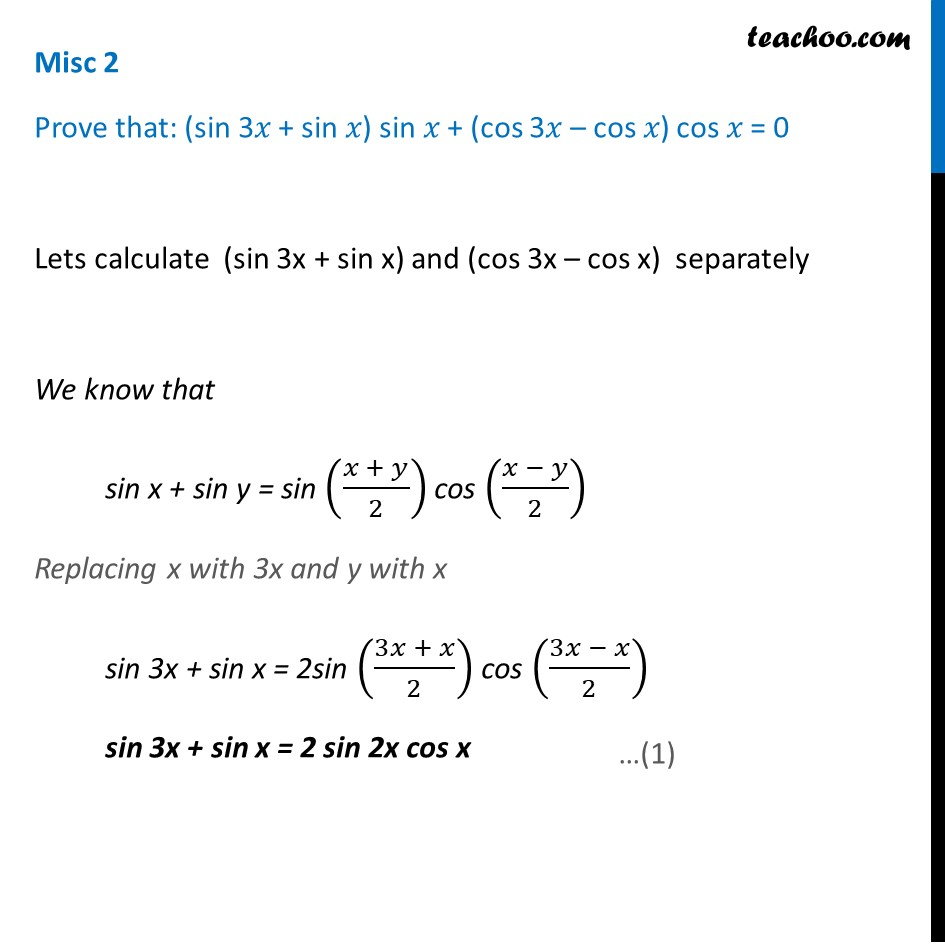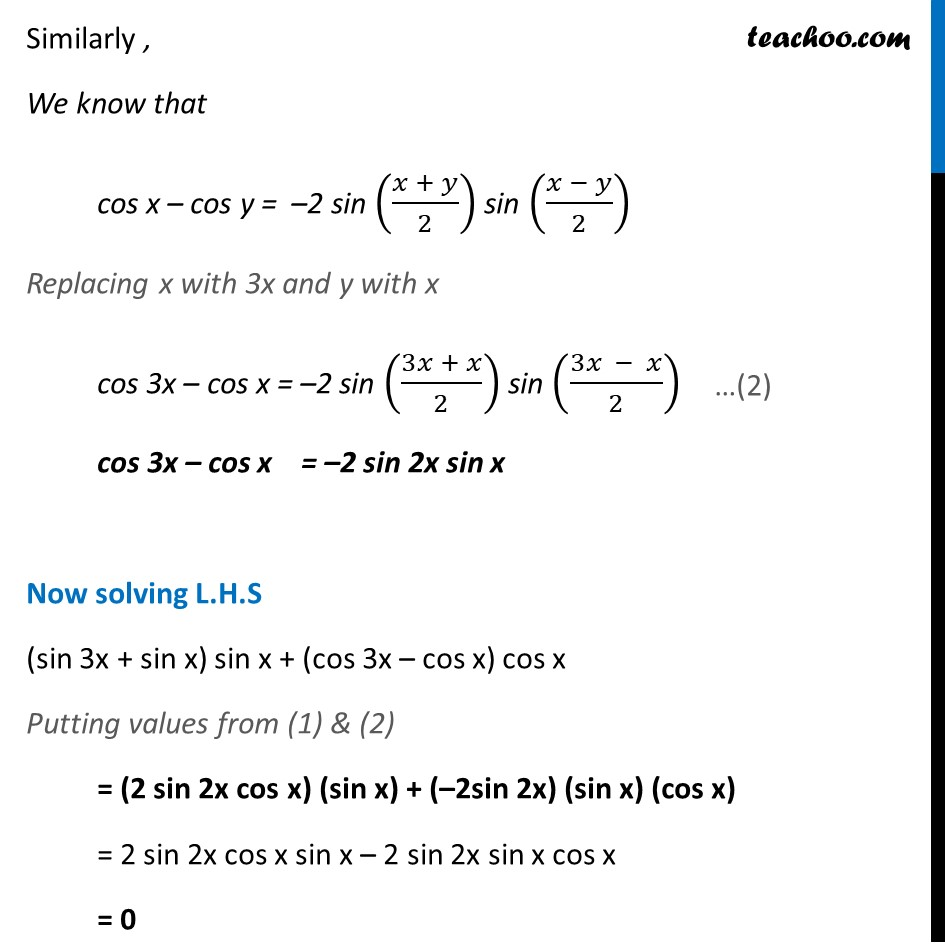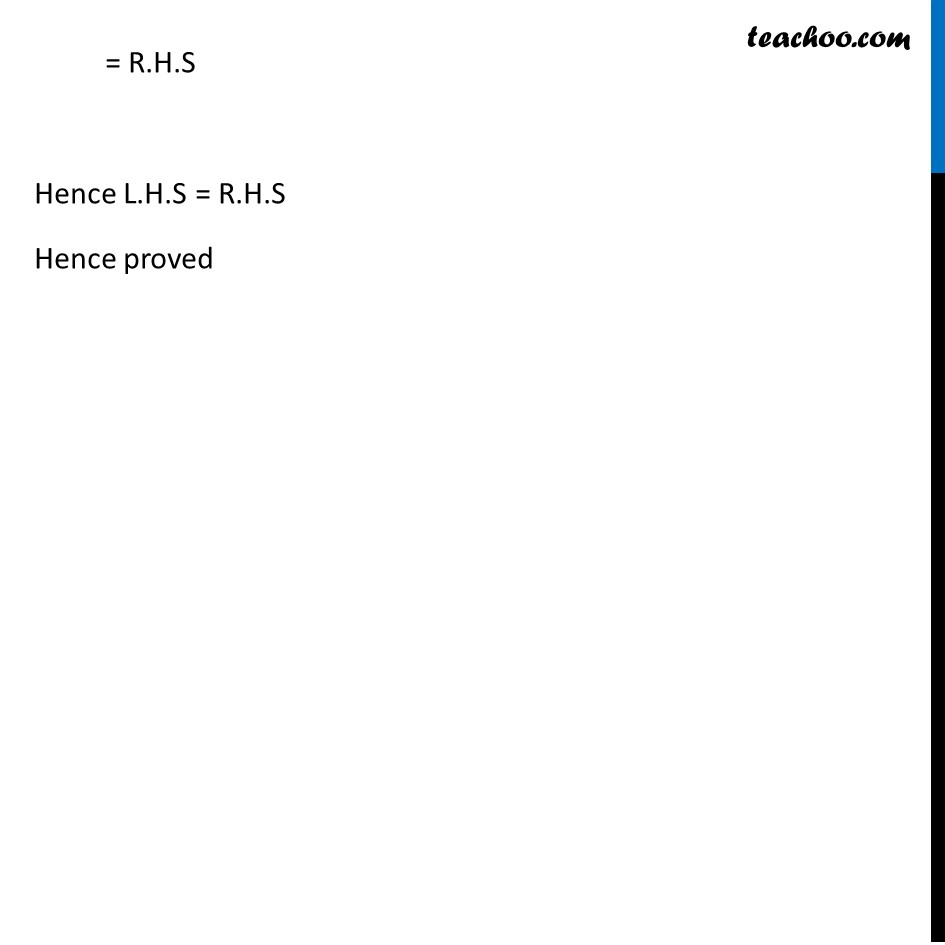Miscellaneous

Chapter 3 Class 11 Trigonometric Functions
Serial order wiseLearn in your speed, with individual attention - Teachoo Maths 1-on-1 Class

### Transcript

Misc 2 Prove that: (sin 3𝑥 + sin 𝑥) sin 𝑥 + (cos 3𝑥 – cos 𝑥) cos 𝑥 = 0 Lets calculate (sin 3x + sin x) and (cos 3x – cos x) separately We know that sin x + sin y = sin ((𝑥 + 𝑦)/2) cos ((𝑥 − 𝑦)/2) Replacing x with 3x and y with x sin 3x + sin x = 2sin ((3𝑥 + 𝑥)/2) cos ((3𝑥 − 𝑥)/2) sin 3x + sin x = 2 sin 2x cos x Similarly , We know that cos x – cos y = –2 sin ((𝑥 + 𝑦)/2) sin ((𝑥 − 𝑦)/2) Replacing x with 3x and y with x cos 3x – cos x = –2 sin ((3𝑥 + 𝑥)/2) sin ((3𝑥 − 𝑥)/2) cos 3x – cos x = –2 sin 2x sin x Now solving L.H.S (sin 3x + sin x) sin x + (cos 3x – cos x) cos x Putting values from (1) & (2) = (2 sin 2x cos x) (sin x) + (–2sin 2x) (sin x) (cos x) = 2 sin 2x cos x sin x – 2 sin 2x sin x cos x = 0 = R.H.S Hence L.H.S = R.H.S Hence proved